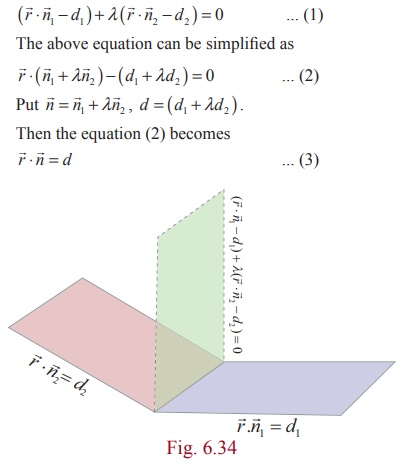Home | | Maths 12th Std | Equation of a plane passing through the line of intersection of two given planes

# Equation of a plane passing through the line of intersection of two given planes

Equation of a plane passing through the line of intersection of two given planes

Equation of a plane passing through the line of intersection of two given planes

### Theorem 6.22

The vector equation of a plane which passes through the line of intersection of the planes### Proof

Consider the equationThe equation (3) represents a plane. Hence (1) represents a plane.

Let1 be the position vector of any point on the line of intersection of the plane. Then1 satisfies both the equations1  = d1  and2  = d2  . So, we haveBy (4) and (5),1 satisfies (1). So, any point on the line of intersection lies on the plane (1). This proves that the plane (1) passes through the line of intersection.

The cartesian equation of a plane which passes through the line of intersection of the planes a1 x + b1y + c1z = d1 and a2x + b2y + c2z = d2 is given by

( a1 b1c1d1) λ (  a2+ b2+ c2= d2) = 0

### Example 6.53

Find the equation of the plane passing through the intersection of the planes(i + j + k )+1 = 0 and(2i - 3+ 5) = 2 and the point (−1, 2,1) .

### Solution

We know that the vector equation of a plane passing through the line of intersection of the planesSince this plane passes through the point (−1, 2,1) , we get λ =3/5 , and hence the required equation of the plane is 11x − 4 y + 20z = 1 .

### Example 6.54

Find the equation of the plane passing through the intersection of the planes 2x + 3y z + 7 = 0 and x + y − 2z + 5 = 0 and is perpendicular to the plane x + y − 3z − 5 = 0 .

### Solution

The equation of the plane passing through the intersection of the planes 2x + 3y z + 7 = 0 and x + y − 2z + 5 = 0 is (2x + 3y z + 7) + λ ( x + y − 2z + 5) = 0 or

(2 + λ ) x + (3 + λ ) y + (−1− 2λ ) z + (7 + 5λ ) = 0

since this plane is perpendicular to the given plane x + y − 3z − 5 = 0 , the normals of these two planes are perpendicular to each other. Therefore, we have

(1)(2 + λ ) + (1)(3 + λ ) + (−3)(−1− 2λ ) z = 0

which implies that λ = −1 .Thus the required equation of the plane is

(2x + 3y z + 7) − ( x + y − 2z + 5) = 0 x + 2 y + z + 2 = 0 .

Study Material, Lecturing Notes, Assignment, Reference, Wiki description explanation, brief detail
12th Mathematics : UNIT 6 : Applications of Vector Algebra : Equation of a plane passing through the line of intersection of two given planes |